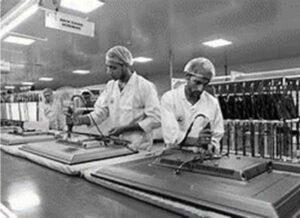Q)   India is competitive manufacturing location due to the low cost of manpower and strong technical and engineering capabilities contributing to higher quality production runs. The production of TV sets in a factory increases uniformly by a fixed number every year. It produced 16000 sets in 6th year and 22600 in 9th year.1) In which year, the production is 29,200 sets?
2) Find the production in the 8th year.
OR
Find the production in first 3 years.
3) Find the difference of the production in 7th year and 4th year.

Ans: Here, we are given that “production of TV sets in a factory increases uniformly by a fixed number every year”. It clearly means that this will form an AP where:

a  = Number of TV sets manufactured in first year

and d = Increase of TV sets every year (that fixed number increasing uniformly every year)

(i) Year of producing 29,200 sets:

We know that the nth term of an AP is given by: Tn  =  a + (n-1) d

Therefore, value of 6th term, T6 = a + (6 – 1) x d = 16000 (given in the question)

a + 5 d = 16000 ……….. (i)

Also, value of 9th term, T9 = a + (9 – 1) x d = 22,600    (given in the question)

a + 8 d = 22600 ……….. (ii)

By subtracting equation (i) from equation (ii), we get:

3 d = 6600 or d = 2200

By substituting value of d in equation (i), we get

a = 16000 – 5 x 2200 or a = 5000

Now, let’s consider the factory will produce 29,200 TV sets in nth year, hence,

Tn  =  a + (n-1) d

29200 =  5000 + (n – 1) x 2200

24200 = (n – 1) 2200

26400 = 2200 x n

or n == 12

Therefore, Factory will produce 24,200 TV sets in 12th year.

(ii) a. Production in the 8th year:

We know that the nth term of an AP is given by: Tn  =  a + (n-1) d

Production of 8th year will be 8th term in the AP….

Therefore, T8 = 5000 + (8 – 1) x 2200 = 5000 + 7 x 2200

= 5000 + 15400 = 20400

Therefore, in 8th year, factory will produce 20,400 TV sets.

OR

(ii) b. Production in first 3 years:

The production in first 3 years will actually be addition of TV sets produced in 1st year, 2nd year and 3rd year.

Or we can sum up first 3 terms:

We know that the sum of n terms of an AP is given by: Sn  =(2a + (n-1) d)

Hence, sum of 3 terms S3  =(2 x 5000 + (3 – 1) 2200) = 21600

Therefore, production of first 3 years will be 21,600 TV sets.

(iii)  Difference of the production in 7th year and 4th years:

We know that the nth term of an AP is given by: Tn  =  a + (n-1) d

Therefore, value of 7th term, T7 = 5000 + (7 – 1) x 2200 = 18200

And, value of 4th term, T4 = 5000 + (4 – 1) x 2200 = 11600

Hence, the difference between the two values = 18200 – 11600 = 6600

Therefore, difference of production in 7th year and 4th years is 6,600 TV sets.

Scroll to Top